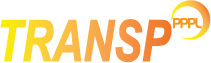# Analysis of the ion temperature

To use the ion temperature, either from a UFILE (PRETI2/EXTTI2) or defined as an analytic profile, set `NLTIPRO=T`. In order to instruct TRANSP to use the provided profile, users also need to set `NLTI2=T`. If this file is not provided, or if `NLTI2=F`, TRANSP will rescale the ion temperature from the electron temperature, as: Ti= GIEFAC x Te

The general dependency is:
Ti(used) = (1 - FIEFAC) (GIEFAC x Te) + FIEFAC x Ti(input)
Thus, if NLTI2=T and the default values `FIEFAC=1, GIEFAC=1` are used, Ti(used)=Ti(input).
Both `FIEFAC` and `GIEFAC` can be made time dependent by using a UFILE, PREFIE/EXTFIE, PREGIE/EXTGIE.

Ion temperature profile data may not cover the plasma edge and/or the plasma core. The following parameters can be used to adjust data in this case:

TIXLIM
maximum value of normalized radius (r/a) where data are valid.
TIFACX
ratio Ti/Te at the edge.
TIDXSW
a linear transition between data and TIFACX*TTe is performed in the interval [TIXLIM, TIXLIM+TIDXSW]. The default value is 0.05.
TIXLIM0
innermost value of normalized radius where the input Ti data are valid. At this radius the difference TDIFF=Ti-Teis evaluated.
TIDXSW0
In the interval [TIXLIM0-TIDXSW0,TIXLIM0] a linear transition between TIFACX0*TDIFF and input data is performed.
TIFACX0
For x≤(TIXLIM0-TIDXSW0) the ion temperature is Ti=Te + TIFACX0*Tdiff.

If the measured temperature is actually an impurity ion temperature (for example from Carbon line), then the additional flag needs to be set: `NLTI2TX=T` (see here documentation on how to deal with input impurity ion temperature).